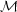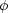1.5. Conditions for their existence: topological criteria

Let us now explore the conditions for the existence of topological defects. It is widely accepted that the final goal of particle physics is to provide a unified gauge theory comprising strong, weak and electromagnetic interactions (and some day also gravitation). This unified theory is to describe the physics at very high temperatures, when the age of the universe was slightly bigger than the Planck time. At this stage, the universe was in a state with the highest possible symmetry, described by a symmetry group G, and the Lagrangian modeling the system of all possible particles and interactions present should be invariant under the action of the elements of G.

As we explained before, the form of the finite temperature effective potential of the system is subject to variations during the cooling down evolution of the universe. This leads to a chain of phase transitions whereby some of the symmetries present in the beginning are not present anymore at lower temperatures. The first of these transitions may be described as GH, where now H stands for the new (smaller) unbroken symmetry group ruling the system. This chain of symmetry breakdowns eventually ends up with SU(3) × SU(2) × U(1), the symmetry group underlying the `standard model' of particle physics.

A broken symmetry system (with a Mexican-hat potential for the Higgs field) may have many different minima (with the same energy), all related by the underlying symmetry. Passing from one minimum to another is included as one of the symmetries of the original group G, and the system will not change due to one such transformation. If a certain field configuration yields the lowest energy state of the system, transformations of this configuration by the elements of the symmetry group will also give the lowest energy state. For example, if a spherically symmetric system has a certain lowest energy value, this value will not change if the system is rotated.

The system will try to minimize its energy and will spontaneously choose one amongst the available minima. Once this is done and the phase transition achieved, the system is no longer ruled by G but by the symmetries of the smaller group H. So, if GH and the system is in one of the lowest energy states (call it S1), transformations of S1 to S2 by elements of G will leave the energy unchanged. However, transformations of S1 by elements of H will leave S1 itself (and not just the energy) unchanged. The many distinct ground states of the system S1, S2 , ... are given by all transformations of G that are not related by elements in H. This space of distinct ground states is called the vacuum manifold and denoted.is the space of all elements of G in which elements related by transformations in H have been identified. Mathematicians call it the coset space and denote it G / H. We then have= G / H.

The importance of the study of the vacuum manifold lies in the fact that it is precisely the topology ofwhat determines the type of defect that will arise. Homotopy theory tells us how to mapinto physical space in a non-trivial way, and what ensuing defect will be produced. For instance, the existence of non contractible loops inis the requisite for the formation of cosmic strings. In formal language this comes about whenever we have the first homotopy group1 ()1, where 1 corresponds to the trivial group. If the vacuum manifold is disconnected we then have0 ()1, and domain walls are predicted to form in the boundary of these regions where the fieldis away from the minimum of the potential. Analogously, if2 ()1 it follows that the vacuum manifold contains non contractible two-spheres, and the ensuing defect is a monopole. Textures arise whencontains non contractible three-spheres and in this case it is the third homotopy group,3(), the one that is non trivial. We summarize this in Table 1.1 .0 ()1disconnected Domain Walls1 ()1 non contractible loops inCosmic Strings2 ()1 non contractible 2-spheres inMonopoles3 ()1 non contractible 3-spheres inTextures